Check the below Online Education NCERT MCQ Questions for Class 8 Maths Chapter 16 Playing with Numbers with Answers Pdf free download. MCQ Questions for Class 8 Maths with Answers were prepared based on the latest exam pattern. We have provided Playing with Numbers Class 8 Maths MCQs Questions with Answers to help students understand the concept very well. https://ncertmcq.com/mcq-questions-for-class-8-maths-with-answers/

Students can also refer to NCERT Solutions for Class 8 Maths Chapter 15 Playing with Numbers for better exam preparation and score more marks.

## Playing with Numbers Class 8 MCQs Questions with Answers

Playing With Numbers Class 8 MCQ Question 1.
The generalised form of the number 52 is
(a) 10 × 5 + 2
(b) 100 × 5 + 2
(c) 10 × 2 + 5
(d) 10 × 5.

Answer: (a) 10 × 5 + 2

MCQ On Playing With Numbers For Class 8 Question 2.
The generalised form of the number 33 is
(a) 10 × 3 + 3
(b) 10 × 3
(c) 3 + 3
(d) 3 × 3 + 3.

Answer: (a) 10 × 3 + 3

Class 8 Maths Chapter 16 MCQ Question 3.
The number 10 × 7 + 5 in usual form is
(a) 57
(b) 75
(c) 55
(d) 77.

Playing With Numbers Class 8 MCQ Questions Question 4.
The number 10 × 2 + 7 in usual form is
(a) 72
(b) 22
(c) 77
(d) 21.

MCQ Questions For Class 8 Maths Playing With Numbers Question 5.
The generalised form of the number 123 is
(а) 1 × 100 + 2 × 10 + 3
(b) 2 × 100 + 3 × 10 + 1
(c) 3 × 100 + 1 × 10 + 2
(d) none of these.

Answer: (а) 1 × 100 + 2 × 10 + 3

Class 8 Playing With Numbers MCQ Question 6.
The generalised form of the number 234 is
(а) 2 × 100 + 3 × 10 + 4
(b) 3 × 100 + 4 × 10 + 2
(c) 4 × 100 + 2 × 10 + 3
(d) none of these.

Answer: (а) 2 × 100 + 3 × 10 + 4

Playing With Numbers MCQ Class 8 Question 7.
The number 3 × 100 + 4 × 10 + 5 in usual form is
(a) 453
(b) 435
(c) 354
(d) 345.

MCQ Of Playing With Numbers Class 8 Question 8.
The number 5 × 100 + 7 × 10 + 9 in usual form is
(a) 795
(b) 759
(c) 579
(d) 597.

Class 8 Maths Playing With Numbers MCQ Question 9.
The number 100 × a + 10 × 6 + c in usual form is
(a) abc
(b) bca
(c) cab
(d) none of these.

MCQs On Playing With Numbers Class 8 Question 10.
The number 100 × b + 10 × c + a in usual form is
(a) bac
(b) bca
(c) cab
(d) cba.

Playing With Numbers Class 8 Extra Questions Question 11.
Find the value of A in the following: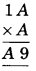(a) 1
(b) 2
(c) 3
(d) 4.

Hint:Playing With Numbers Class 8 Extra Questions With Answers Question 12.
Find the value of A, B in the following: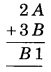(a) 5, 6
(b) 5, 5
(c) 6, 5
(d) 6, 6.

Hint: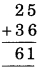MCQ Questions For Class 8 Maths Chapter 16 Question 13.
Find the value of A, B in the following: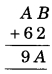(a) 3, 1
(b) 1, 1
(c) 3, 3
(d) 1, 3.

Hint: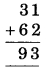MCQ Playing With Numbers Class 8 Question 14.
Find the value of A, B in the following: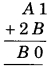(a) 6, 9
(b) 6, 6
(c) 9, 6
(d) 9, 9

Hint:Playing With Numbers Class 8 MCQ With Answers Question 15.
Find the values of A, B in the following: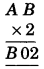(a) 5, 1
(b) 5, 5
(c) 1, 1
(d) 1, 5.

Hint:Question 16.
Which of the following numbers is divisible by 2 ?
(a) 19
(b) 27
(c) 99
(d) 50.

Hint:
In 19, 27, 99, one’s digit is not divisible by 2.

Question 17.
Which of the following numbers is divisible by 2 ?
(a) 179
(b) 235
(c) 500
(d) 673.

Hint:
In 500, one’s digit is divisible by 2.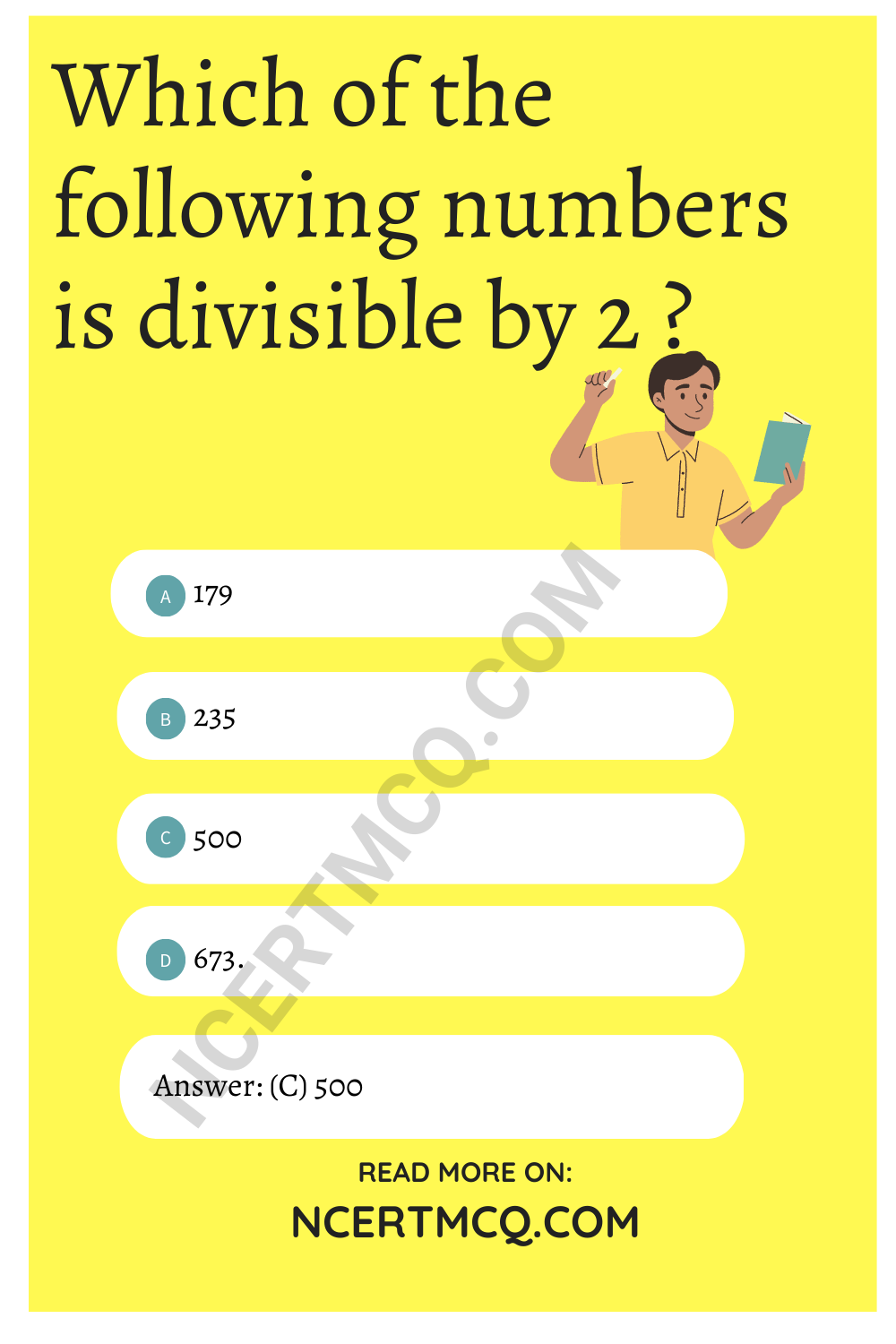Question 18.
Which of the following numbers is not divisible by 2 ?
(a) 200
(b) 40
(c) 66
(d) 83.

Hint:
In 83, one’s digit is not divisible by 2.

Question 19.
Which of the following numbers is not divisible by 2 ?
(a) 54
(b) 37
(c) 60
(d) 98.

Hint:
In 37, one’s digit is not divisible by 2.

Question 20.
Which of the following numbers is not divisible by 2 ?
(a) 120
(b) 244
(c) 888
(d) 179.

Hint:
In 179, one’s digit is not divisible by 2.

Question 21.
If the number 1 x 8 is divisible by 3, then x is equal to
(a) 0 or 3 or 6 or 9
(b) 4
(c) 5
(d) 7.

Answer: (a) 0 or 3 or 6 or 9
Hint:
1 + 0 + 8 = 9
1 + 3 + 8 = 12;
9, 12, 15, 18 each is divisible by 3

Question 22.
If the number 9 y 7 is a multiple of 3, then y =
(a) 4
(b) 3
(c) 6
(d) 2 or 5 or 8.

Answer: (d) 2 or 5 or 8.
Hint:
9 + 2 + 7 = 18
9 + 5 + 7 = 21;
9 + 8 + 7 = 24.
each of 18, 21 and 24 is divisible by 3.

Question 23.
If the three-digit number 4 3 x is divisible by 9, what is the value of x ?
(a) 1
(b) 2
(c) 3
(d) 4.

Hint:
4 + 3 + 2 = 9 which is divisible by 9.

Question 24.
If the three-digit number x 2 7 is divisible by 9, then the value of x is
(a) 8
(b) 7
(c) 6
(d) 9.

Hint:
9 + 2 + 7 = 18 is divisible by 9.

Question 25.
If the three-digit number 6 y 8 is divisible by 9, then the value of y is
(a) 1
(b) 2
(c) 3
(d) 4.

Hint:
6 + 4 + 8 = 18 is divisible by 9.

Question 26.
Which of the following numbers is not divisible by 3 ?
(a) 123
(b) 132
(c) 231
(d) 134.

Hint:
1 + 3 + 4 = 8 is not divisible by 3.

Question 27.
Which of the following numbers is not divisible by 3 ?
(a) 234
(b) 243
(c) 324
(d) 457.

Hint:
4 + 5 + 7 = 16 is not divisible by 3.

Question 28.
Which of the following numbers is divisible by 3 ?
(a) 145
(b) 237
(c) 709
(d) 400.

Hint:
2 + 3 + 7 = 12 is divisible by 3.

Question 29.
Which of the following numbers is divisible by 9 ?
(a) 234
(b) 334
(c) 444
(d) 434.

Hint:
2 + 3 + 4 = 9 is divisible by 9.

Question 30.
Which of the following numbers is not divisible by 9 ?
(a) 135
(b) 351
(c) 513
(d) 247.

Hint:
2 + 4 + 7 = 13 is not divisible by 9.

Question 31.
Which of the following numbers is divisible by 5 ?
(a) 125
(b) 127
(c) 731
(d) 339.

Hint:
One’s digit of 125 is 5.

Question 32.
Which of the following numbers is divisible by 5 ?
(a) 120
(b) 233
(c) 348
(d) 447.

Hint:
One’s digit of 120 is 0.

Question 33.
Which of the following numbers is not divisible by 5 ?
(a) 120
(b) 235
(c) 355
(d) 477.

Hint:
In 477, one’s digit is neither 0 nor 5.

Question 34.
Which of the following numbers is divisible by 10?
(a) 40
(b) 35
(c) 57
(d) 69.

Hint:
In 40, one’s digit is 0.

Question 35.
Which of the following numbers is divisible by 10?
(a) 235
(b) 3400
(c) 278
(d) 1277.

Hint:
In 3400, one’s digit is 0.

Question 36.
Which of the following numbers is not divisible by 10?
(a) 120
(b) 340
(c) 760
(d) 1246.

Hint:
In 1246, one’s digit is not 0.

Question 37.
The usual form of 100 × 7 + 10 × 1 + 8 is:
(a) 108
(b) 708
(c) 718
(d) 170

Hint:
100 × 7 + 10 × 1 + 8
= 700 + 10 + 8
= 718

Question 38.
Which of the following numbers are not divisible by 5?
(a) 20
(b) 125
(c) 122
(d) 200

Hint:
To be completely divisible by 5, the number should have 0 or 5 at it’s one’s digit place.

Question 39.
Which of the following numbers are divisible by 10?
(a) 99
(b) 45
(c) 110
(d) 75

Hint:
If any number has 0 at its one’s digit place, then it is divisible by 10.
Hence, 110/10 = 11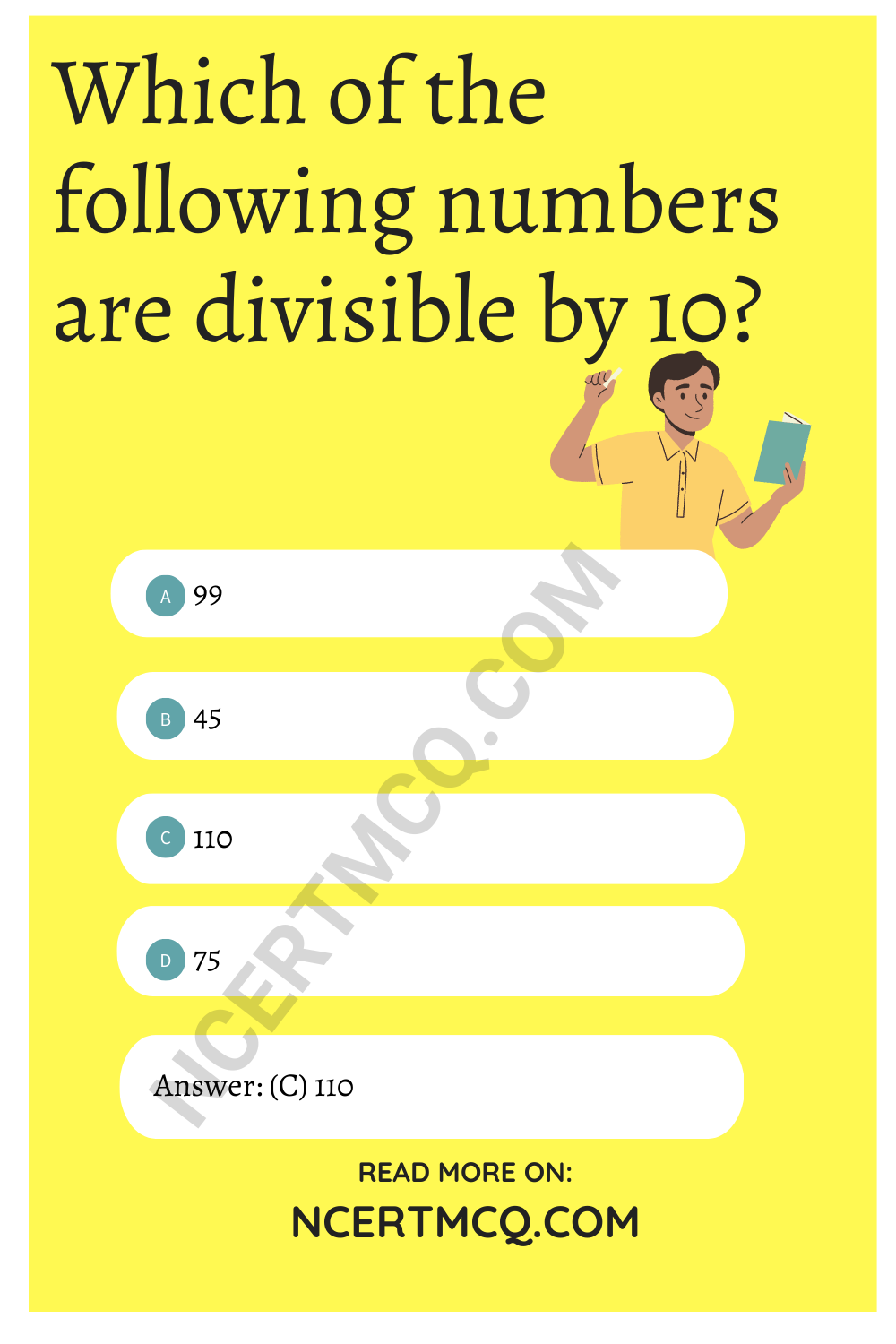Question 40.
Which of the following are divisible by 2?
(a) 98
(b) 99
(c) 101
(d) 121

Hint:
If one’s digit of any number is even number, then the number is completely divisible by 2.
Hence, 98/2 = 49

Question 41.
If a number is divisible 9, then it is divisible by:
(a) 6
(b) 7
(c) 3
(d) 11

Hint:
If 99 is divisible by 9 then it is also divisible by 3.
99/9 = 11 and 99/3 = 33

Question 42.
If the three digit number 24x is divisible by 9, the value of x is:
(a) 3
(b) 7
(c) 1
(d) None of the above

Hint:
If 24x is divisible by 9, then:
2 + 4 + x = 6 + x is also divisible by 9.
It is possible only when, 6 + x = 9, 18, …
Since x is single digit, therefore, 6 + 3 = 9
x = 3

Question 43.
The number 2146587 is divisible by:
(a) 7
(b) 3
(c) 11
(d) None of the above

Hint:
2146587 = 2 + 1 + 4 + 6 + 5 + 8 + 7 = 33
Since, 33 is divisible by 3, hence 2146587 is divisible by 3.

Question 44.
The general form of abc is:
(a) 100a + 10b + c
(b) 100b + 10c + a
(c) 100c + 10a + b
(d) None of the above

Answer: (a) 100a + 10b + c

Question 45.
The generalised form of 129 is:
(a) 100 + 90 + 2
(b) 100 + 20 + 9
(c) 100 + 2 + 9
(d) None of the above

Answer: (b) 100 + 20 + 9

Question 46.
The number 15287 is divisible by:
(a) 3
(b) 7
(c) 9
(d) None of the above